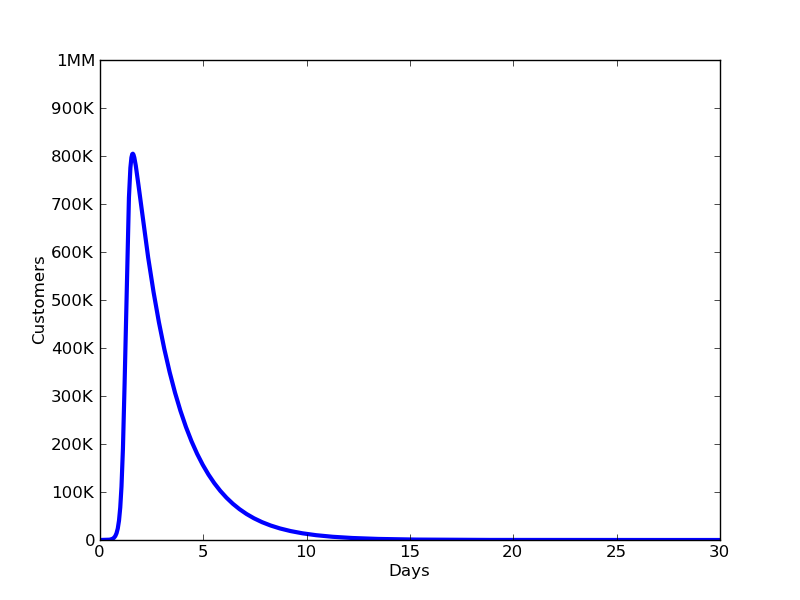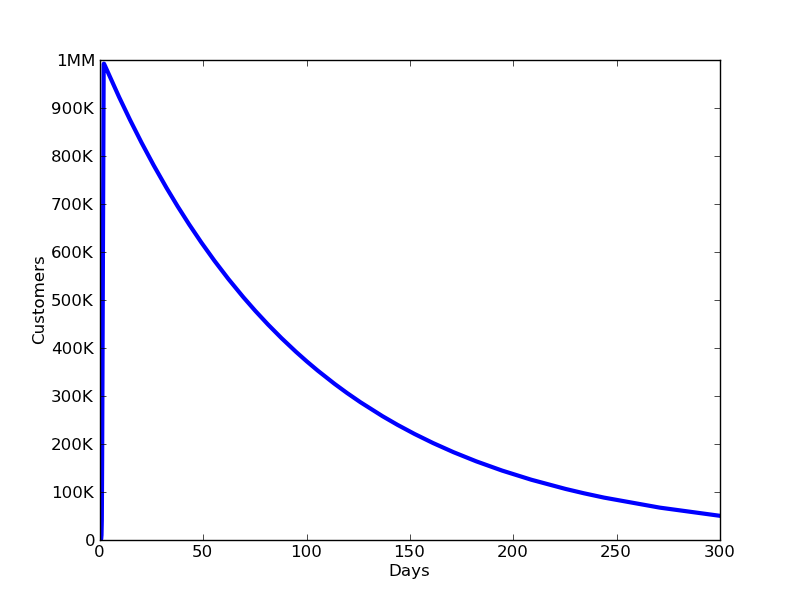# A Better Mathematical Model of Viral Marketing

This is the second part of a four part series of blog posts on viral marketing. In part 1, I discuss the faulty assumptions in the current models of viral marketing. In part 3, I show the weird dynamics of viral marketing in a growing market. In part 4, I'll discuss the effects of returning customers.

Current models of viral marketing for the business community rely on faulty assumptions. As a result, these models fail to reflect real world examples.

So how can the business community build a more realistic model of viral marketing? How do you know which factors (e.g. viral coefficient, time scale, churn, market size) are most important? Fortunately, there is a rich history of literature on mathematical models for viral growth (and decline), dating all the way back to 1927. These models rigorously treat viral spread, churn, market size, and even the change in the market size and the possibility that former customers return. Obviously, nobody was thinking of making a YouTube video or an iPhone app go viral back when phones didn't even have rotary dials. These models are of the viral spread of … viruses!

The Model:

The classic SIR model of the spread of disease is by Kermack and McKendrick. (Sorry I couldn’t link to the original paper. You can buy it for \$54.50 here -- blame the academicpublishingindustry). I’ve applied this model to viral marketing by drawing analogies between a disease and a product. The desired outcomes are very different, but the math is the same.

Kermack and McKendrick divide the total population of the market, $N$, into three subpopulations.

• $S$ - The number of people susceptible to the disease (potential customers)
• $I$ - The number of people who are infected with the disease (current customers)
• $R$ - The number of people who have recovered from the disease (former customers).

These three subpopulations change in number over time. The population of potential customers become current customers as a result of successful invitations. Current customers become former customers if they decide to stop using the product. For simplicity, I’ll treat the total market size, $N = S + I + R$, as static and former customers as immune (for now). The parameters that govern spread of disease are:

• $β$ - The infection rate (sharing rate)
• $γ$ - The recovery rate (churn rate)

Assume that current customers, $I$, and potential customers, $S$, communicate with each other at an average rate that is proportional to their numbers (as governed by the Law of Mass Action). This gives $βSI$ as the number of new customers, per unit time, due to word of mouth or online sharing. As the number of new customers grows by $βSI$, the number of potential customers shrinks by the same number. This plays the same role that the “viral coefficient” does in Skok’s model, but accounts for the fact that conversion rates on sharing slow down when the fraction of people who have already tried the product gets large. It also does away with the concept of "cycle time". Instead, it accounts for the average time it takes to share something and the average frequency at which people share by putting a unit of time into the denominator of $β$. Thus, $β$ represents the number of successful invitations per current customer per potential customer per unit time (i.e. hour, day, week). I propose that this is a more robust definition of viral coefficient than the one used by Ries and Skok because modeling viral sharing as an average rate accounts for the following realities:

• Customers do not share in synchronous batches.
• Each user has a different timeframe for trying a product, learning to love it, and sharing it with friends. Rather than assuming that they all have the same cycle time, $β$ represents an average rate of sharing.
• Users might invite others when first trying a product or after they’ve used it for quite a while.

In this model, current customers become former customers at a rate defined by the parameter $γ$. That is, $γ$ is the fraction of current customers who become former customers in a unit of time. It has the dimensions of inverse time $(1/t)$, and $1/γ$ represents the average time a user remains a user. So, if $γ = 1\%$ of users lost per day, then the average length of time a user remains active is 100 days.

The differential equations governing viral spread are:

• $dS/dt = -βSI$
• $dI/dt = βSI - γI$
• $dR/dt = γI$

Examining the Equations:

These are non-linear differential equations that cannot be solved to produce convenient, insight yielding formulas for $S(t)$, $I(t)$, and $R(t)$. What they lack in convenient formulas, they make up for with more interesting dynamics (especially when considering changing market sizes and returning customers). You can still learn a lot by examining them and integrating them numerically. Let’s assume that $t=0$, represents the launch of a new product. Initially, at least the founding team uses the product and represent the initial customer base, $I(0)$. The initial number of former customers, $R(0)$, is zero and the rest of the people in the market are potential customers, $S(0)$.

The first thing to note is that there will be a growing customer base $(dI/dt > 0)$ as long as:

$βS/γ > 1$

That is, viral growth will occur as long as the addressable market size, $S(0)$, and sharing rate, $β$, are sufficiently large compared to the churn, $γ$. This model shows that with a big enough market, you can go viral even with a small $β$ as long as your churn is also small enough (consistent with the Pinterest example described in part 1). This model also shows that the effects of churn cannot be ignored, even in very early viral growth.

If at $t=0$, $S$ is very close to $N$, then $βS/γ$ is approximately $βN/γ$. Thus, if $βN/γ > 1$, initial growth will occur and if $βN/γ < 1$, the customer base will not grow. This is sometimes called the “basic reproductive number” in epidemiology literature. It is essentially what Eric Ries calls the “viral coefficient” although it depends on market size and churn as well as the viral sharing rate. It is approximately the average number of new customers each early customer will invite during the entire time that they remain a customer, which is $1/γ$. However, in the case that viral growth does occur, $βN/γ$ rapidly ceases to represent the number of customers that each customer invites.

Another thing you can see by examining the equations is that if you ignore the change in the market size (an approximation that makes sense for short lived virality, such as with a YouTube video), the customer base always goes to zero at long times unless you have zero churn. Once the number of current customers reaches a peak where $dI/dt = 0$ at $I = N - γ/β$, the rate of change in the number of current customers becomes negative and the number of customers eventually reaches zero. This is consistent with the data provided in the Mashable post on the half-lives of Twitter vs. YouTube content. Again, note the key role that churn has in determining the peak number of customers.

Examples:

We can gain more insight from these equations by numerically integrating them. For these examples, the unit of time used to define $β$ and $γ$ is one day, though the choice is arbitrary. I’ve given values of $β$ as $βN$ to create better correspondence with Ries’ concept of viral coefficient -- If at $t=0$, $S(0)$ is approximately $N$, $βN$ is approximately the number of new customers each existing customer begets per day.

With the parameters:

• $N = 1$ million people in the market
• $βN = 10$ invites per current user per day
• $γ = 50\%$ of customers lost per day
• $I(0) = 10$ current customersnumerically integrating the equations given above yields the following for how the number of customers changes for the first 30 days: This shows a traffic pattern similar to that of a popular Twitter link where traffic quickly spikes and then dies down as people tire of looking at it. (In the case of visiting a webpage, a “customer” can be defined as a visitor).For a smaller churn rate, $γ = 1\%$ of customers lost per day, we see the following for the growth and decline in the number of customers over 300 days: This shows how even for low values of churn, without new potential customers joining the market, or former customers returning, the customer base always diminishes after reaching it's peak. Also note how a smaller churn rate allows us to reach a higher peak in traffic.

So how can viral growth be sustained? For that, you need to consider how the change in the market size affects viral marketing, which I’ll examine in part 3.

(For another fun example of how to apply the SIR model, see my post on the Mathematics of the Walking Dead.)

TLDR: A better definition of “viral coefficient” is successful invitations per existing user per potential user per unit time. But market size and customer churn are just as, if not more, important than viral coefficient. Viral growth in a static market is unsustainable unless you have absolutely zero churn.

Image Credit.